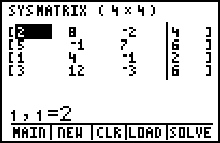# Software, OS Updates and Apps

## Polynomial Root Finder and Simultaneous Equation SolverExtend the benefits and functions of the TI-86 to your calculator.

Polynomial Root Finder and Simultaneous Equation Solver 2.00 56 2
Guidebooks
Polynomial Root Finder & Simultaneous Equation guidebook for TI-83 Plus / TI-84 Plus (English) View: 344
Polynomial Root Finder & Simultaneous Equation guidebook for TI-83 Plus / TI-84 Plus (Suomalainen) View: 265
Polynomial Root Finder & Simultaneous Equation guidebook for TI-83 Plus / TI-84 Plus (Deutsch) View: 208
Polynomial Root Finder & Simultaneous Equation guidebook for TI-83 Plus / TI-84 Plus (Espanol) View: 373
Polynomial Root Finder & Simultaneous Equation guidebook for TI-83 Plus / TI-84 Plus (Francais) View: 377

## Features Summary

### Polynomial Root Finder

• Enter polynomials up to and including order (degree) 10
• Easy to use POLY MODE screen to set up all options
• Display roots as fractions or decimals for many roots
• Choose to display only Real roots for 2nd and 3rd degree polynomials
• For polynomials of degree 4 and higher, roots display in Complex format
• Store polynomials to Y= for graphing and evaluation
• Verify a root is the zero of the polynomial function by storing roots in Real format.

### Simultaneous Equation Solver

• Enter systems of equations with up to 10 equations and 10 unknowns
• Easy to use SIMULT MODE screen to set up all options
• Displays unique solution, infinite solution, and no solution
• Store coefficient matrix, augmented matrix, and the solution
• Displays Reduced Row Echelon form

• The Polynomial Root Finder and Simultaneous Equation Solver App is available for the TI-83 Plus, TI-83 Plus Silver Edition, TI-84 Plus, and TI-84 Plus Silver Edition Graphing Calculators. The Polynomial Root Finder App and the Simultaneous Equation Solver Apps are each available separately for the TI-89, TI-89 Titanium, TI-92 Plus, and Voyage 200 Graphing Calculators.